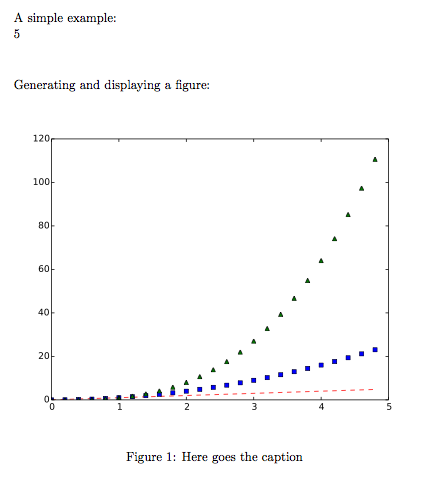### Running Python code in a LaTeX document

I am currently working on a LaTeX document in which the content is still in a very fluid phase and  the figures change often. I usually have a Python script that prepares the figures and saves them as PDFs. To iterate faster, I wanted to insert Python code directly into LaTeX, executing the code when the document is compiled.

Googling around I found that I was not the first one to want this. There is this LaTeX package with this github repo. The package provides a new environment (python) within which you can write arbitrary Python code. The code is executed when the document is compiled and the output is inserted into the document.

I try it and it worked like a charm. It was even printing the traceback in red when there was an error in the script. You can do things like this:

\documentclass[a4paper]{book}

\usepackage[pdftex]{graphicx}
\usepackage{python}

\begin{document}

A simple example:

\begin{python}
print(2+3)
\end{python}

\vspace{1cm}
Generating and displaying a figure:

\begin{python}
import numpy as np
import matplotlib.pyplot as plt

# evenly sampled time at 200ms intervals
t = np.arange(0., 5., 0.2)

# red dashes, blue squares and green triangles
plt.plot(t, t, 'r--', t, t**2, 'bs', t, t**3, 'g^')
plt.savefig('fig1.pdf', format='pdf')
print(r"""
\begin{figure}[htbp]
\centering
\includegraphics[width=12cm]{fig1.pdf}
\caption{Here goes the caption}
\label{fig:comparison}
\end{figure}
""")

\end{python}

\end{document}


and the output is:But as the document grew, the LaTeX compilation time increased dramatically. The problem: Python scripts are executed on each compilation even if they have not changed.

I have forked the repo and hacked the code. In the new version, python scripts are only executed if needed. Using a helper python script (runpy.py) to avoid encoding differences between terminals, UTF-8 is fully supported. Additionally, you can select the python binary to use and choose to save auxiliary files in a subfolder to avoid poluting the main folder. These options are explained in the README. Check the code is in this github repo.

I am not really a LaTeX programmer and therefore I am sure that there a lot of things to be improved. Feel free to send patches, suggestions and comments.